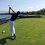# All ramps are not equal

I have a very tiny block that slides down a ramp in the Cartesian plane from point $$a$$ to point $$b$$. This ramp can be modelled by the function $$f(x)$$ for $$x_a\leq x \leq x_b$$. For all values of c such that $$a<c \leq b$$, $$f(c)<f(a)$$. If the block manages to slide from point $$a$$ to $$b$$. Prove that the maximum velocity of the block at point $$b$$ is achieved when $$f(x)$$ is a straight line from $$a$$ to $$b$$ if friction is present.

I will post the proof for this if no one has within 48 hours.Note by Trevor Arashiro
5 years, 2 months ago

This discussion board is a place to discuss our Daily Challenges and the math and science related to those challenges. Explanations are more than just a solution — they should explain the steps and thinking strategies that you used to obtain the solution. Comments should further the discussion of math and science.

When posting on Brilliant:

• Use the emojis to react to an explanation, whether you're congratulating a job well done , or just really confused .
• Ask specific questions about the challenge or the steps in somebody's explanation. Well-posed questions can add a lot to the discussion, but posting "I don't understand!" doesn't help anyone.
• Try to contribute something new to the discussion, whether it is an extension, generalization or other idea related to the challenge.

MarkdownAppears as
*italics* or _italics_ italics
**bold** or __bold__ bold
- bulleted- list
• bulleted
• list
1. numbered2. list
1. numbered
2. list
Note: you must add a full line of space before and after lists for them to show up correctly
paragraph 1paragraph 2

paragraph 1

paragraph 2

[example link](https://brilliant.org)example link
> This is a quote
This is a quote
    # I indented these lines
# 4 spaces, and now they show
# up as a code block.

print "hello world"
# I indented these lines
# 4 spaces, and now they show
# up as a code block.

print "hello world"
MathAppears as
Remember to wrap math in $$ ... $$ or $ ... $ to ensure proper formatting.
2 \times 3 $2 \times 3$
2^{34} $2^{34}$
a_{i-1} $a_{i-1}$
\frac{2}{3} $\frac{2}{3}$
\sqrt{2} $\sqrt{2}$
\sum_{i=1}^3 $\sum_{i=1}^3$
\sin \theta $\sin \theta$
\boxed{123} $\boxed{123}$

Sort by:

As the end points are fixed and are separated by a verical distance of $h$ (say), by work-kinetic energy theorem (and a little simplification), we have, $\frac{v_b^2}{2}=gh-\mu_kg s$ where $\mu_k$ is the coefficient of kinetic friction and $s$ is the length of the horizontal section of the ramp.

As the points $a$ and $b$ are fixed, so is $s$. Hence, speed of the block remains constant.

PS: This was a nice fallacy which our teacher had discussed.

- 5 years, 2 months ago

It must be "$\mu_k g s$"

- 5 years, 2 months ago

- 5 years, 2 months ago

That's the same start to my proof, but when you say horizontal section of the ramp, are you referring only to the length of the ramp in the x-direction or the total length of the ramp? And another thing to think about: at different angles, while u is constant, the frictional force is greater for flatter sections of the ramp, so does your equation still work for curved ramps?

- 5 years, 2 months ago

I meant the length of the horizontal section only.

Yes, this is valid for curved ramps as well. Here's the proof:

Consider a point on the ramp. Let the tengent at this point make an angle $\theta$ with the horizontal. Then, if $dW$ denotes the work done by friction over a path length $ds$, $dW=-\mu_kmg\cos \theta ds$ But, note that $ds\, \cos \theta=dx$. Hence, $dW=-\mu_kmg dx\\\implies W=-\mu_kmg(x_b-x_a)$ Assuming $x_b>x_a$

So, all ramps are actually equal ;)

- 5 years, 2 months ago

What if slope of the curve is less than or equal to $\mu_{k}$?

- 5 years, 2 months ago

The question states that the body manages to slide to point $b$. So, kinetic friction will always act.

Plus, your objection makes sense if you said that $\mu_s \geq \tan \theta$. The inequality $\mu_k \geq \tan \theta$ makes no difference to the motion of the block.

- 5 years, 2 months ago

Not too difficult of a proof but it does require calc (at least the way I did it... which I pray is right)

- 5 years, 2 months ago